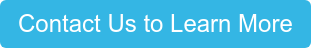Prior to the “new normal,” commercial real estate investors threw around cap rate numbers with impunity. At the beginning of the year we were seeing triple net deals transacting with cap rates in the 4.0% to 5.0% range and multifamily buildings trading in the 5.0% to 6.0% range. These deals represent the best in class properties, but where are cap rates headed?

Right now, that remains to seen, but while we wait for things to settle, let’s look at how cap rates are calculated. The table below shows the basic formula to calculate a cap rate.

 RO = (M × RM) + ((1 – M) × RE) RO Overall Rate M Loan to Value RM Mortgage Constant RE Equity Dividend Rate

The easiest way to understand the formula is to look at an example, so in the table below are our assumptions.

 Property Information List Price \$      500,000 Net Operating Income \$         35,000 Capital Stack Equity (or down payment) \$      125,000 25% Loan \$      375,000 75% Total \$      500,000 Loan Assumptions Loan Amount \$      375,000 Interest Rate 7.00% Term (mo) 360 Amortization (mo) 360 Monthly Payment \$2,494.88 Mortgage Constant 7.98%

The only other assumption we need at this point is to determine the Equity Dividend Rate or expected return to an investor (pre-tax). For this example, we are going to assume the investor is looking for a 10% return on their equity.  If we assume this is a purchase and you put down \$125,000 as your down payment, then your equity would be \$125,000.  Your loan to value, or LTV, would be 75%, calculated by dividing your loan amount by the list price.

RO = (0.75 × .0798) + (0.25 × 0.10)

RO = 0.05985 + .0250

RO = .08485

RO = 8.5% (rnd)

Based on the loan and equity assumptions, we calculated an 8.5% cap rate. Now from our previous blog “How to Calculate Cap Rate and Determine NOI,” we know we can divide the NOI by the cap rate to determine the estimated market value of property.

Value = NOI ÷ RO

Value = \$35,000 ÷ 8.5%

Value = \$411,765 (rnd)

We also know that we can take the NOI divided by the purchase price to determine the asking cap rate, which in this case is 7.0%. Based on calculated cap rate of 8.5%, we feel the property given the loan and equity assumptions, is worth 17.6% less than the list price. Now while this formula is only one way of determining a cap rate it is a useful tool and is included in most narrative commercial appraisals.

But back to the original question, where are cap rates headed?

In my opinion, higher as I think, at least for short period of time, we will see an increase in interest rates for commercial properties and a reduction in the LTVs.  Both factors play a significant role in the calculation of cap rates as we have learned.

To learn more about Small Balance Commercial concepts and strategies, see our "Small Balance Commercial" blog category.Topics: Small Balance Commercial